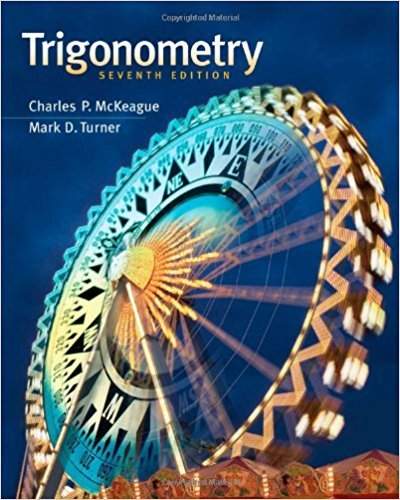×
Log in to StudySoup
Get Full Access to Trigonometry - 7 Edition - Chapter 4.1 - Problem A
Join StudySoup for FREE
Get Full Access to Trigonometry - 7 Edition - Chapter 4.1 - Problem A

Already have an account? Login here
×
Reset your password

# How do we use the unit circle to graph the function y sinISBN: 9781111826857 186

## Solution for problem a Chapter 4.1

Trigonometry | 7th Edition

• Textbook Solutions
• 2901 Step-by-step solutions solved by professors and subject experts
• Get 24/7 help from StudySoup virtual teaching assistantsTrigonometry | 7th Edition

4 5 1 249 Reviews
13
0
Problem a

How do we use the unit circle to graph the function y sin x?

Step-by-Step Solution:
Step 1 of 3

Elementary Statistical Methods Notes: January 11-15, 2016 Chapter 1: Intro to Statistics Section 1.1 Statistics is the science of collecting, describing, and interpreting data. There are two types of Statistics:  Descriptive Statistics (Chapters 2-4)  Inferential Statistics (Chapters 8-11) (Chapters 5-7 cover probability)  Chapter 5: Probability  Chapter 6: Discrete probability distribution  Chapter 7: Continuous probability distribution Descriptive Statistics includes the collection, presentation, and description of sample data (histograms, bar graphs, pie charts, etc…) Inferential Statistics consists of procedures to make inferences or conclusions about population characteristics from the info contained in the sample. A variable is a

Step 2 of 3

Step 3 of 3

##### ISBN: 9781111826857

The answer to “How do we use the unit circle to graph the function y sin x?” is broken down into a number of easy to follow steps, and 14 words. Trigonometry was written by and is associated to the ISBN: 9781111826857. Since the solution to a from 4.1 chapter was answered, more than 269 students have viewed the full step-by-step answer. The full step-by-step solution to problem: a from chapter: 4.1 was answered by , our top Math solution expert on 12/27/17, 07:46PM. This textbook survival guide was created for the textbook: Trigonometry, edition: 7. This full solution covers the following key subjects: . This expansive textbook survival guide covers 85 chapters, and 4184 solutions.

Unlock Textbook Solution

Enter your email below to unlock your verified solution to:

How do we use the unit circle to graph the function y sin